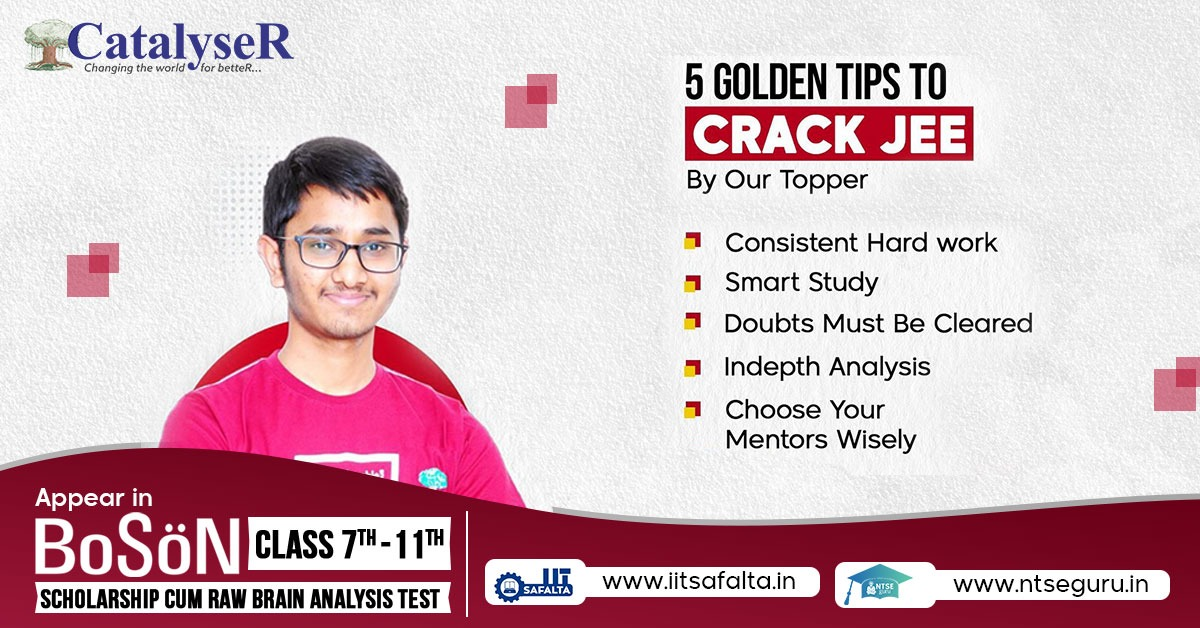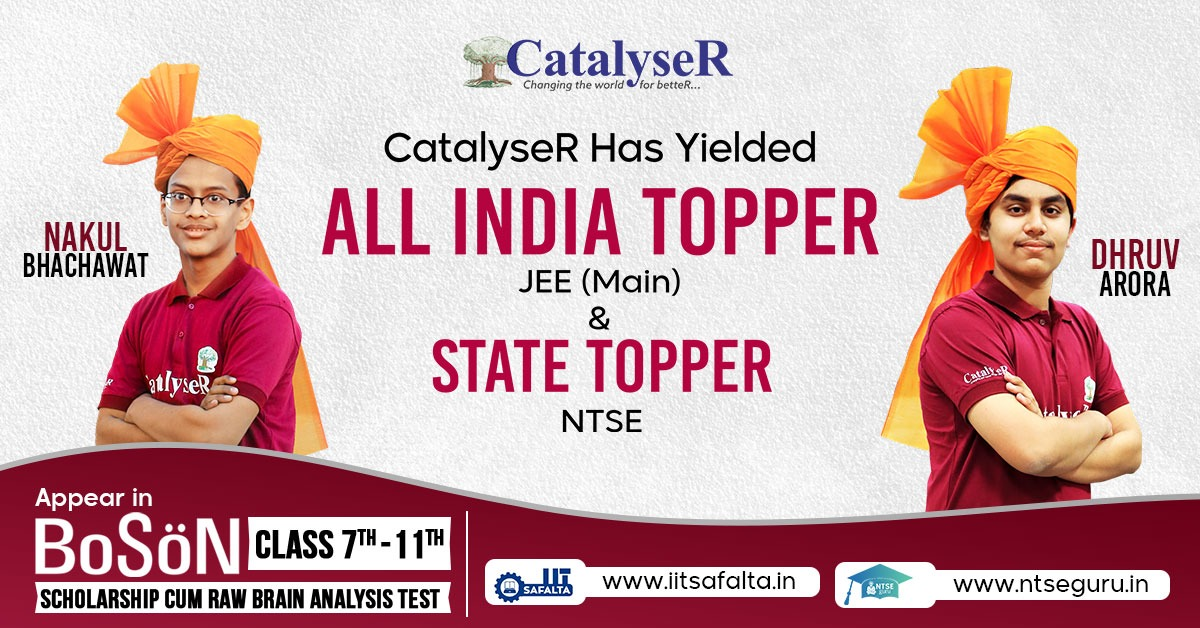# Study Tips to Prepare Mathematics for JEE MainStudy Tips to Prepare Mathematics for JEE Main

It's crucial to prepare thoroughly for the JEE Mains. Mathematics is a really very important subject that can give you an edge over others.

Even if Math, Chemistry, and Physics are weighted equally in the exam, Mathematics is more important than the others because it is the deciding factor in the end. Why?

The foundation of Physics and Chemistry is built on mathematics. Nearly all of the numerical problems in Physics and Chemistry are based on quadratic equations, differential equations, and calculus.The IIT JEE Math syllabus features both difficult as well as simple topics. Permutations and combinations, quadratic equations, trigonometry, probability, straight lines and circles, and differential calculus are some of the simple topics which will boost your confidence.

At CatalyseR the faculties analyse various factors and derive conclusions which help students sharpen their axe. 74% students in JEE (Mains) & 22% conversion in JEE (Advanced) against National Ratio Of 20% in JEE (Mains) & 1% in JEE (Advanced).

If we talk about the last year results of the CatalyseR students, we can see that aspirants got the highest marks in the mathematics section.

<img src="https://catalyser.in/public/img/blog/boson.jpeg" alt="" width="100%"/>

## Important Topics in Mathematics for JEE Mains

### Algebra

Among the scoring topics of the JEE, algebra is one of the most important.

In addition to being an interesting topic, algebra also requires a fast computation speed as well as a clear understanding of the concept to get outstanding scores. Some of the topics that aspirants should cover in algebra are:

1. Differential Equations

2. Progressions and Series

3. Sets, Relations, and Functions

5. Permutation and Combination

6. Complex Numbers

7. Matrices and Determinants

8. Binomial Theorem

9. Probability

### Calculus

Calculus is one of the biggest topics in contemporary mathematics and is regarded as one of the key topics for IIT JEE preparation.

It includes two principal topics: Differential and Integral Calculus, which include the fundamental theorem of calculus.

Calculus includes studying techniques and applying them to problem-solving. In JEE Mathematics, problems based on theory as well as those based on practical applications are of equal frequency.

It is very important to know calculus for the JEE Exams as a high weightage of approximately 40% is assigned to this topic.

### Coordinate Geometry

The relatively simple topic of coordinate geometry is the study of geometrical shapes and curves on a graph. It is one of the topics in which you can score full marks.

The topics covered in coordinate geometry include straight lines, hyperbolas, parabolas, ellipses, and circles. It's one of the most scoring topics in the mathematics section of the JEE Exams and you can score full marks in it.

### Trigonometry

Trigonometry is regarded as one of the most significant topics. The sides and angles of a triangle are linked, and this relation is studied in trigonometry. The IIT JEE Exam covers a wide range of topics, ranging from basic trigonometric functions to applied trigonometry.

Trigonometric ratios and trigonometric functions are frequently asked in JEE Mains and other exams. You must be proficient with the fundamental principles of trigonometry because several competitive exams include numerous questions on this topic.

## Recommended Books for JEE Main Mathematics Preparation

Book Name

Publications

Maths for Class XI & XII

R.D. Sharma

Maths for Class XI & XII

R.S. Agarwal

Mathematics Volumes

Cengage Publication, Arihant Publication

Previous Years’ Question Paper

Disha Publication, Arihant Publication

Class XI & XII

NCERT

### Final Note - Tips to Prepare Mathematics for JEE Main

Using this strategy will help you prepare for mathematics in IIT JEE Mains. To enhance your knowledge and track your performance, you can join the Test Series session and Crash Course at CatalyseR.

CatalyseR is offering Online courses for students willing to learn through online mode.

Students can prepare for their exams with the assistance of a CatalyseR. We provide video lectures, online practice tests, and online study material with their online courses.

Register for BoSon Test now!